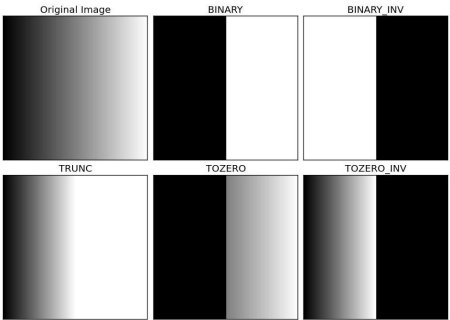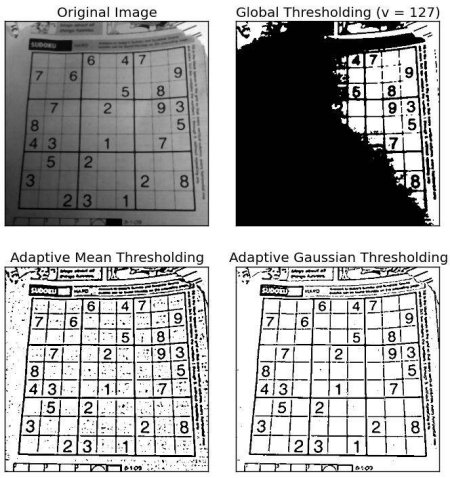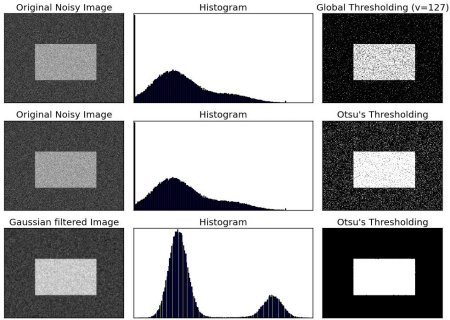# 目标

• 你会学到简单阈值法，自适应阈值法，以及 Otsu 阈值法(俗称大津法)等。

## 简单阈值法

import cv2 as cv
import numpy as np
from matplotlib import pyplot as plt
ret,thresh1 = cv.threshold(img,127,255,cv.THRESH_BINARY)
ret,thresh2 = cv.threshold(img,127,255,cv.THRESH_BINARY_INV)
ret,thresh3 = cv.threshold(img,127,255,cv.THRESH_TRUNC)
ret,thresh4 = cv.threshold(img,127,255,cv.THRESH_TOZERO)
ret,thresh5 = cv.threshold(img,127,255,cv.THRESH_TOZERO_INV)
titles = ['Original Image','BINARY','BINARY_INV','TRUNC','TOZERO','TOZERO_INV']
images = [img, thresh1, thresh2, thresh3, thresh4, thresh5]
for i in xrange(6):
plt.subplot(2,3,i+1),plt.imshow(images[i],'gray')
plt.title(titles[i])
plt.xticks([]),plt.yticks([])
plt.show()## 自适应阈值

Block Size-它决定了计算阈值的窗口区域的大小。

C-它只是一个常数，会从平均值或加权平均值中减去该值。

import cv2 as cv
import numpy as np
from matplotlib import pyplot as plt
img = cv.medianBlur(img,5)
ret,th1 = cv.threshold(img,127,255,cv.THRESH_BINARY)
cv.THRESH_BINARY,11,2)
cv.THRESH_BINARY,11,2)
titles = ['Original Image', 'Global Thresholding (v = 127)',
images = [img, th1, th2, th3]
for i in xrange(4):
plt.subplot(2,2,i+1),plt.imshow(images[i],'gray')
plt.title(titles[i])
plt.xticks([]),plt.yticks([])
plt.show()## Otsu 二值化

import cv2 as cv
import numpy as np
from matplotlib import pyplot as plt
# 全局阈值
ret1,th1 = cv.threshold(img,127,255,cv.THRESH_BINARY)
# Otsu 阈值
ret2,th2 = cv.threshold(img,0,255,cv.THRESH_BINARY+cv.THRESH_OTSU)
# 经过高斯滤波的 Otsu 阈值
blur = cv.GaussianBlur(img,(5,5),0)
ret3,th3 = cv.threshold(blur,0,255,cv.THRESH_BINARY+cv.THRESH_OTSU)
# 画出所有的图像和他们的直方图
images = [img, 0, th1,
img, 0, th2,
blur, 0, th3]
titles = ['Original Noisy Image','Histogram','Global Thresholding (v=127)',
'Original Noisy Image','Histogram',"Otsu's Thresholding",
'Gaussian filtered Image','Histogram',"Otsu's Thresholding"]
for i in xrange(3):
plt.subplot(3,3,i*3+1),plt.imshow(images[i*3],'gray')
plt.title(titles[i*3]), plt.xticks([]), plt.yticks([])
plt.subplot(3,3,i*3+2),plt.hist(images[i*3].ravel(),256)
plt.title(titles[i*3+1]), plt.xticks([]), plt.yticks([])
plt.subplot(3,3,i*3+3),plt.imshow(images[i*3+2],'gray')
plt.title(titles[i*3+2]), plt.xticks([]), plt.yticks([])
plt.show()## Otsu 二值化原理

$$\sigma_w^2(t) = q_1(t)\sigma_1^2(t)+q_2(t)\sigma_2^2(t)$$ 其中： $$\begin{eqnarray} q_1(t) = \sum_{i=1}^{t} P(i) \quad & \quad q_2(t) = \sum_{i=t+1}^{I} P(i) \ \mu_1(t) = \sum_{i=1}^{t} \frac{iP(i)}{q_1(t)} \quad & \quad \mu_2(t) = \sum_{i=t+1}^{I} \frac{iP(i)}{q_2(t)} \ \sigma_1^2(t) = \sum_{i=1}^{t} [i-\mu_1(t)]^2 \frac{P(i)}{q_1(t)} \quad & \quad \sigma_2^2(t) = \sum_{i=t+1}^{I} [i-\mu_2(t)]^2 \frac{P(i)}{q_2(t)} \end{eqnarray}$$

img = cv.imread('noisy2.png',0)
blur = cv.GaussianBlur(img,(5,5),0)
# 找到归一化直方图还有累计分布函数
hist = cv.calcHist([blur],,None,,[0,256])
hist_norm = hist.ravel()/hist.max()
Q = hist_norm.cumsum()
bins = np.arange(256)
fn_min = np.inf
thresh = -1
for i in xrange(1,256):
p1,p2 = np.hsplit(hist_norm,[i]) # 概率
q1,q2 = Q[i],Q-Q[i] # 类别总和
b1,b2 = np.hsplit(bins,[i]) # 权重
# f 找到均值与方差
m1,m2 = np.sum(p1*b1)/q1, np.sum(p2*b2)/q2
v1,v2 = np.sum(((b1-m1)**2)*p1)/q1,np.sum(((b2-m2)**2)*p2)/q2
# 计算最小函数
fn = v1*q1 + v2*q2
if fn < fn_min:
fn_min = fn
thresh = i
# 用 OpenCV 函数的 otsu'阈值
ret, otsu = cv.threshold(blur,0,255,cv.THRESH_BINARY+cv.THRESH_OTSU)
print( "{} {}".format(thresh,ret) )


## 其他资源

1、Digital Image Processing, Rafael C. Gonzalez

## 练习

1、Otsu 二值化有很多的优化方法，你可以尝试搜索相关资料后自己实现。